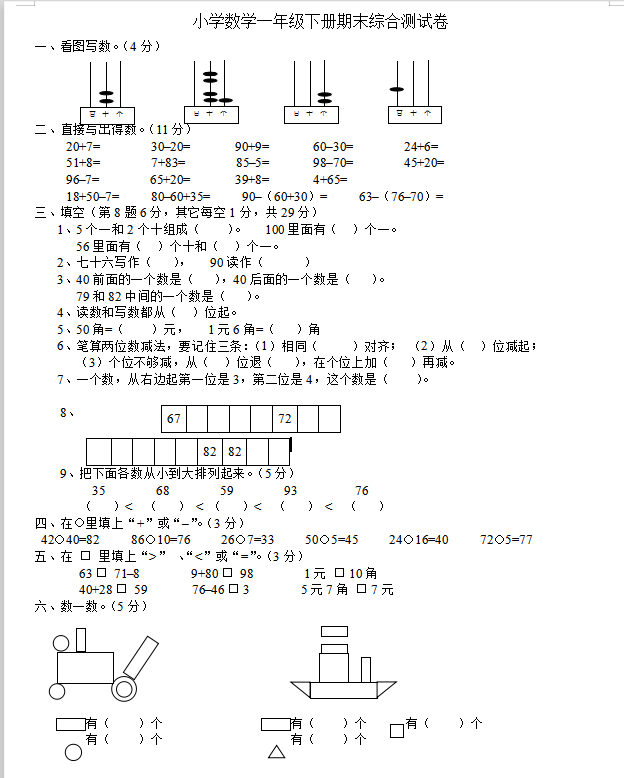20+7=        30–20=       90+9=       60–30=        24+6=

51+8=        7+83=        85–5=       98–70=        45+20=

96–7=        65+20=       39+8=       4+65=